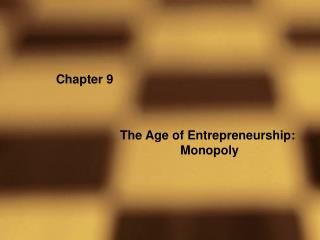# Chapter 9 - PowerPoint PPT PresentationDownload PresentationChapter 9

Chapter 9
Download Presentation## Chapter 9

- - - - - - - - - - - - - - - - - - - - - - - - - - - E N D - - - - - - - - - - - - - - - - - - - - - - - - - - -
##### Presentation Transcript

1. Chapter 9 The Age of Entrepreneurship: Monopoly

2. Figure 9.1 The Average and Marginal Cost Functions for Our Entrepreneur’s Firm

3. Figure 9.2 Linear Market Demand Function

4. Marginal Revenue Demand: p = A - bq Start at point q on the demand curve: R = pq = (A – bq)q = Aq – bq2 Increase output to q + Dq (a little more output) R’ = p (q + Dq) = (A – b(q + Dq)) (q + Dq) The change in Revenue from q to q + Dq is DR = R – R’ = (Aq – bq2) - (A – b(q + Dq)) (q + Dq) Which simplifies to DR = Dq(A – 2bq – bDq) Marginal revenue is the change in Revenue divided by the change in q DR/Dq = (A – 2bq – bDq) as Dq approaches 0 => A – 2bq

5. Figure 9.3 Marginal Revenue and Demand

6. Figure 9.4 Optimal Price and Quantity

7. The Socially Optimal Price Price A B p* MC C D E ps G H F K L J I Demand q* qs Quantity MR

8. Solved Problem 9.5 • AC = 10; MC = 10; q = 100 – ½ p; p = 200 – 2q • Calculate the socially optimal price and quantity of water • What would the price and quantity be if he charged as a Monopolist • Set demand = MC • 200 – 2q = 10  q = 95 (substitute into demand curve) p = \$10 • Consumer Surplus = ½ (190)(95) = 9025; Producer Surplus = 0 • b) Set MR = MC • 200 – 4q = 10  q = 47.5 (substitute into demand curve) p = \$105 • Consumer Surplus = 2256.25; Producer Surplus = 4512.5 • Overall surplus falls to 6768.75

9. Profitability: Never Be Profitable

10. Profitability: Normal Profit

11. Profitability: Extra-Normal Profit